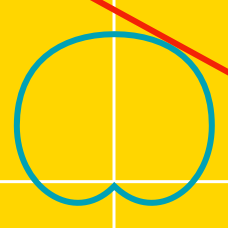Calculus

# Polar Equations - Surface Area

Find the area of the surface formed by revolving the curve $$r = 5 \sin\theta$$ from $$\theta = 0$$ to $$\theta = \frac{\pi}{2}$$ about the $$x$$-axis.

Find the surface area generated when the curve $$r = e^{\theta}$$ from $$\theta = 0$$ to $$\theta = \frac{\pi}{2}$$ is revolved about the $$y$$-axis.

Find the area of the surface formed by revolving the curve $$r = 5 \sin\theta$$ from $$\theta = 0$$ to $$\theta = \frac{\pi}{2}$$ about the $$y$$-axis.

Find the area of the surface formed by revolving the curve $$r = 3 \cos(\theta)$$ from $$\theta = 0$$ to $$\theta = \frac{\pi}{2}$$ about the $$x$$-axis.

Find the area of the surface formed by revolving the curve $$r = 5 \cos{\theta}$$ from $$\theta = 0$$ to $$\theta = \frac{\pi}{2}$$ about the $$y$$-axis.

×